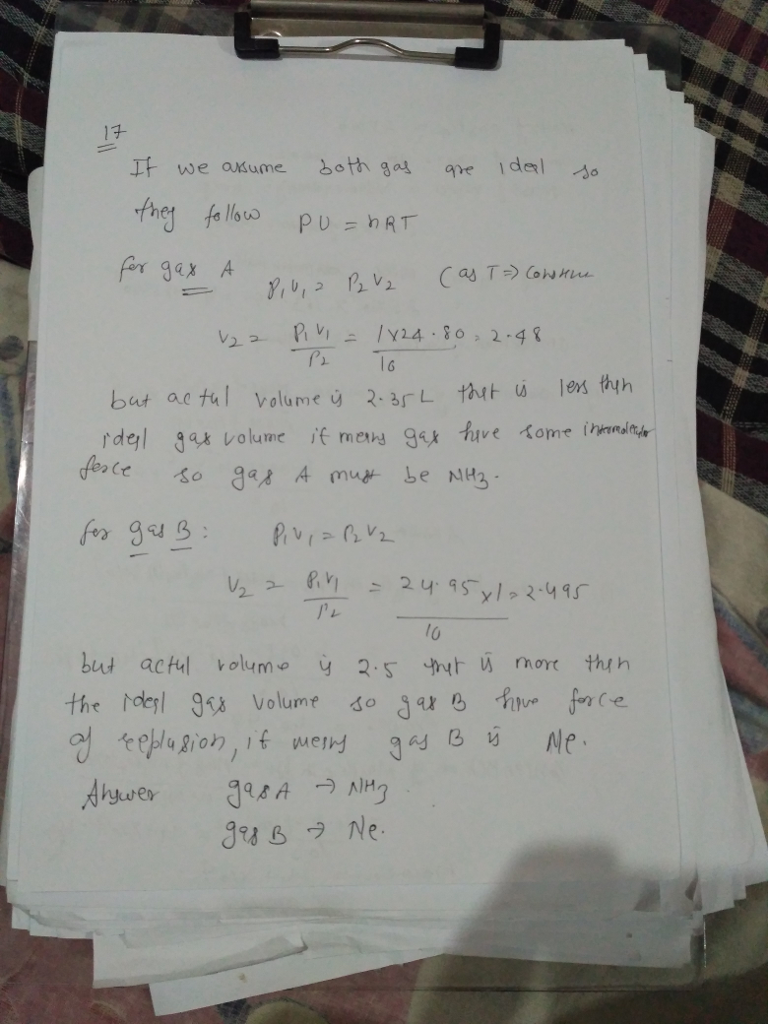# Homework Solution: Two gases have the following P-V relationships at 300 K: P = 1.00 bar P = 10.0…

Two gases have the following P-V relationships at 300 K: P = 1.00 bar P = 10.0 bar one mole of Gas A V= 24.80 L/mole V= 2.35 L/mol one mole of Gas B V= 24.95 L/mole V= 2.51L/mol One of these gases is Ne and the other is NH_3. Identify each and explain your reasoning.